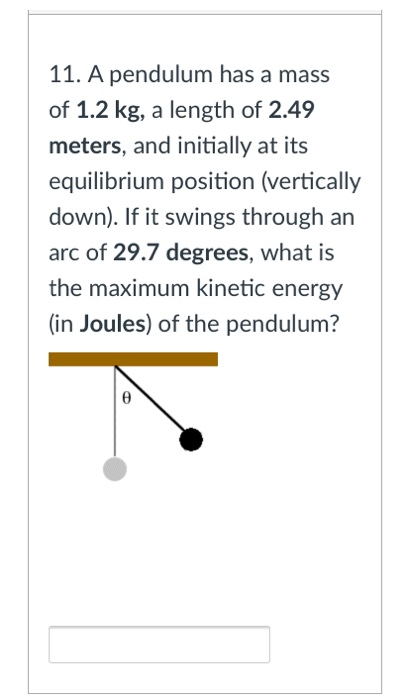# Question Solved1 Answer11. A pendulum has a mass of 1.2 kg, a length of 2.49 meters, and initially at its equilibrium position (vertically down). If it swings through an arc of 29.7 degrees, what is the maximum kinetic energy (in Joules) of the pendulum?Transcribed Image Text: 11. A pendulum has a mass of 1.2 kg, a length of 2.49 meters, and initially at its equilibrium position (vertically down). If it swings through an arc of 29.7 degrees, what is the maximum kinetic energy (in Joules) of the pendulum?
More
Transcribed Image Text: 11. A pendulum has a mass of 1.2 kg, a length of 2.49 meters, and initially at its equilibrium position (vertically down). If it swings through an arc of 29.7 degrees, what is the maximum kinetic energy (in Joules) of the pendulum?